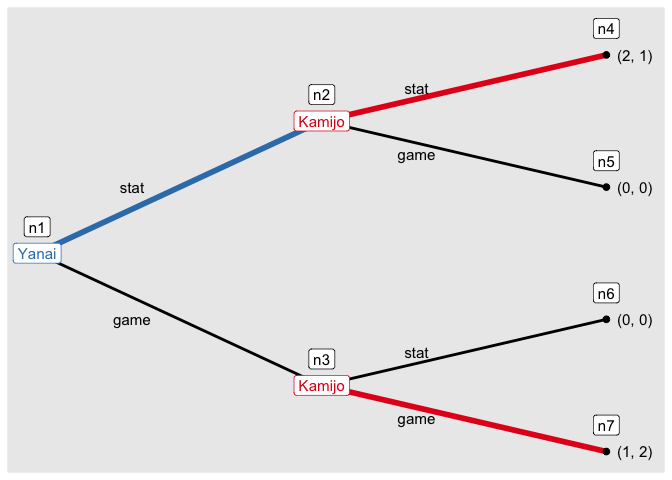## Overview

The goal of rgamer is to help students learn Game Theory using R. The functions prepared by the package not only solve basic games such as two-person normal-form games but also provides the users with visual displays that highlight some aspects of the games — payoff matrix, best response correspondence, etc. In addition, it suggests some numerical solutions for games of which it is difficult — or even seems impossible — to derive a closed-form analytical solution.

## Installation

You can install the development version from GitHub with:

# install.packages("remotes")
remotes::install_github("yukiyanai/rgamer")

or

# install.packages("devtools")
devtools::install_github("yukiyanai/rgamer")

## Examples

library(rgamer)

### Example 1

An example of a normal-form game (prisoner’s dilemma).

• Player: Kamijo, Yanai
• Strategy: (Stays silent, Betrays), (Stays silent, Betrays)
• Payoff: (-1, 0, -3, -2), (-1, -3, 0, -2)

First, you define the game by normal_form():

game1 <- normal_form(
players = c("Kamijo", "Yanai"),
s1 = c("Stays silent", "Betrays"),
s2 = c("Stays silent", "Betrays"),
payoffs1 = c(-1,  0, -3, -2),
payoffs2 = c(-1, -3,  0, -2))

You can specify payoffs for each cell of the game matrix as follows.

game1b <- normal_form(
players = c("Kamijo", "Yanai"),
s1 = c("Stays silent", "Betrays"),
s2 = c("Stays silent", "Betrays"),
cells = list(c(-1, -1), c(-3,  0),
c( 0, -3), c(-2, -2)),
byrow = TRUE)

Then, you can pass it to solve_nfg() function to get the table of the game and the Nash equilibrium.

s_game1 <- solve_nfg(game1, show_table = FALSE)
#> Pure-strategy NE: [Betrays, Betrays]
s_game1$table Yanai strategy Stays silent Betrays Kamijo Stays silent -1, -1 -3, 0^ Betrays 0^, -3 -2^, -2^ ### Example 2 An example of a coordination game. • Player: Kamijo, Yanai • Strategy: (Stag, Hare), (Stag, Hare) • Payoff: (10, 8, 0, 7), (10, 0, 8, 7) Define the game by normal_form(): game2 <- normal_form( players = c("Kamijo", "Yanai"), s1 = c("Stag", "Hare"), s2 = c("Stag", "Hare"), payoffs1 = c(10, 8, 0, 7), payoffs2 = c(10, 0, 8, 7)) Then, you can pass it to solve_nfg() function to get NEs. Set mixed = TRUE to find mixed-strategy NEs well. s_game2 <- solve_nfg(game2, mixed = TRUE, show_table = FALSE) #> Pure-strategy NE: [Stag, Stag], [Hare, Hare] #> Mixed-strategy NE: [(7/9, 2/9), (7/9, 2/9)] #> The obtained mixed-strategy NE might be only a part of the solutions. #> Please examine br_plot (best response plot) carefully. For a 2-by-2 game, you can plot the best response correspondences as well. s_game2$br_plot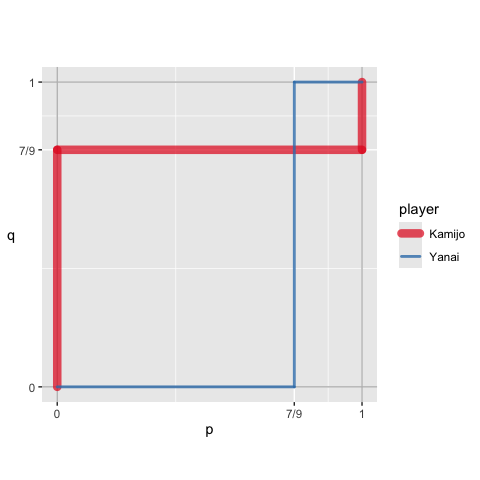### Example 3

An example of a normal-form game:

• Player:
• Strategy:
• Payoff:

You can define a game by specifying payoff functions as character vectors using normal_form():

game3 <- normal_form(
players = c("A", "B"),
payoffs1 = "-x^2 + (28 - y) * x",
payoffs2 = "-y^2 + (28 - x) * y",
par1_lim = c(0, 30),
par2_lim = c(0, 30),
pars = c("x", "y"))

Then, you can pass it to solve_nfg(), which displays the best response correspondences by default.

s_game3 <- solve_nfg(game3)
#> approximated NE: (9.3, 9.3)
#> The obtained NE might be only a part of the solutions.
#> Please examine br_plot (best response plot) carefully.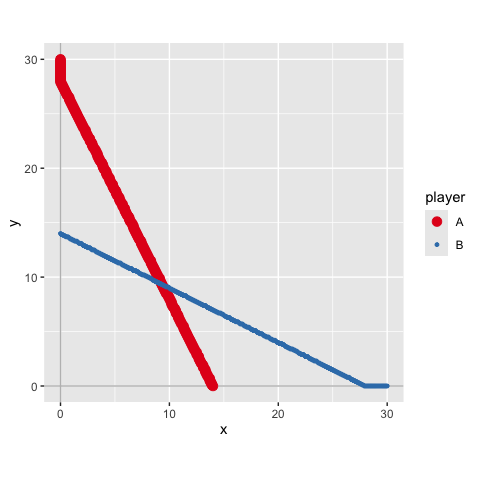### Example 4

An example of a normal-form game:

• Player:
• Strategy:
• Payoff:

You can define a normal-form game by specifying payoffs by R functions.

f_x <- function(x, y, a, b) {
-x^a + (b - y) * x
}
f_y <- function(x, y, s, t) {
-y^s + (t - x) * y
}
game4 <- normal_form(
players = c("A", "B"),
payoffs1 = f_x,
payoffs2 = f_y,
par1_lim = c(0, 30),
par2_lim = c(0, 30),
pars = c("x", "y"))

Then, you can approximate a solution numerically by solve_nfg(). Note that you need to set the parameter values of the function that should be treated as constants by arguments cons1 and cons2, each of which accepts a named list. In addition, you can suppress the plot of best responses by plot = FALSE.

s_game4 <- solve_nfg(
game = game4,
cons1 = list(a = 2, b = 28),
cons2 = list(s = 2, t = 28),
plot = FALSE)
#> approximated NE: (9.3, 9.3)
#> The obtained NE might be only a part of the solutions.
#> Please examine br_plot (best response plot) carefully.

You can increase the precision of approximation by precision, which takes a natural number (default is precision = 1).

s_game4b <- solve_nfg(
game = game4,
cons1 = list(a = 2, b = 28),
cons2 = list(s = 2, t = 28),
precision = 3)
#> approximated NE: (9.333, 9.333)
#> The obtained NE might be only a part of the solutions.
#> Please examine br_plot (best response plot) carefully.You can extract the best response plot with NE marked as follows.

s_game4b\$br_plot_NE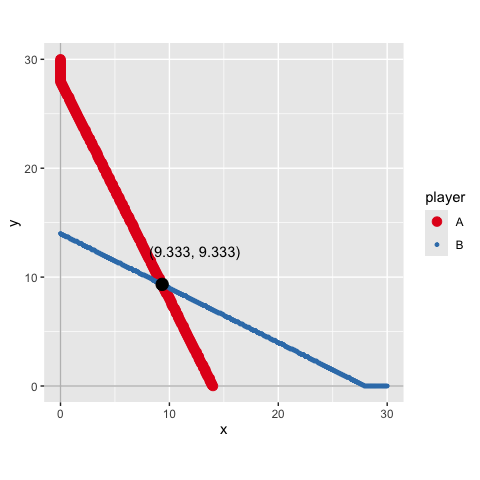## Example 5

You can define payoffs by R functions and evaluate them at some discretized values by setting discretize = TRUE. The following is a Bertrand competition example:

func_price1 <- function(p, q) {
if (p < q) {
profit <- p
} else if (p == q) {
profit <- 0.5 * p
} else {
profit <- 0
}
profit
}

func_price2 <- function(p, q){
if (p > q) {
profit <- q
} else if (p == q) {
profit <- 0.5 * q
} else {
profit <- 0
}
profit
}

game5 <- normal_form(
payoffs1 = func_price1,
payoffs2 = func_price2,
pars = c("p", "q"),
par1_lim = c(0, 10),
par2_lim = c(0, 10),
discretize = TRUE)

Then, you can examine the specified part of the game.

s_game5 <- solve_nfg(game5, mark_br = FALSE)

Player 2

strategy 0 2 4 6 8 10
Player 1 0 0, 0 0, 0 0, 0 0, 0 0, 0 0, 0
2 0, 0 1, 1 2, 0 2, 0 2, 0 2, 0
4 0, 0 0, 2 2, 2 4, 0 4, 0 4, 0
6 0, 0 0, 2 0, 4 3, 3 6, 0 6, 0
8 0, 0 0, 2 0, 4 0, 6 4, 4 8, 0
10 0, 0 0, 2 0, 4 0, 6 0, 8 5, 5

## Example 6

You can draw a tree of an extensive form game.

game6 <- extensive_form(
players = list("Yanai",
rep("Kamijo", 2),
rep(NA, 4)),
actions = list(c("stat", "game"),
c("stat", "game"), c("stat", "game")),
payoffs = list(Yanai = c(2, 0, 0, 1),
Kamijo = c(1, 0, 0, 2)),
direction = "right")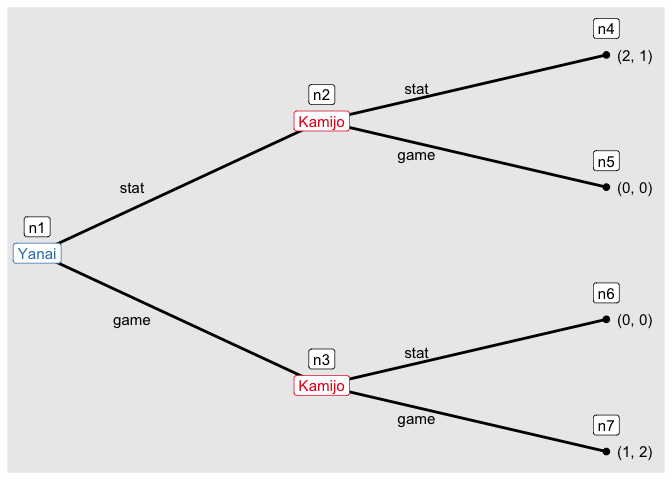And you can find the solution of the game by solve_efg().

s_game6 <- solve_efg(game6)
#> backward induction: [(stat), (stat, game)]

Then, you can see the path played under a solution by show_path().

show_path(s_game6)